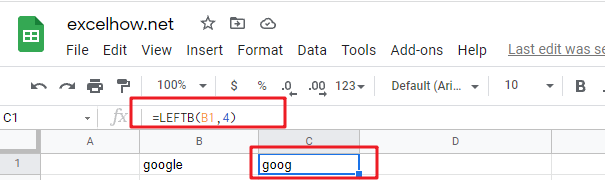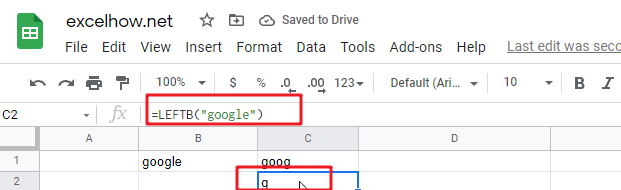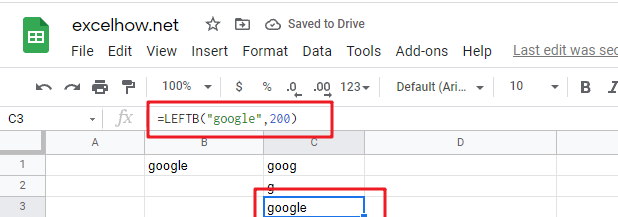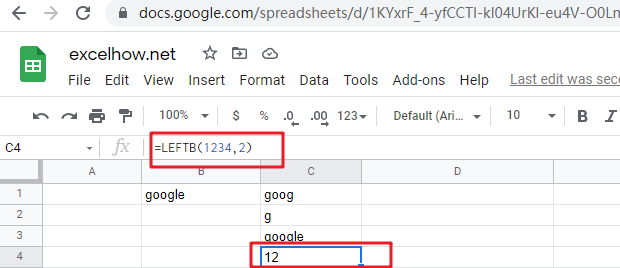# ExcelHow

This post will guide you how to use Google Sheets LEFTB function with syntax and examples.

## Description

The Google Sheets LEFTB function returns a substring (a specified number of the bytes) from a text string, starting from the left portion.

The LEFTB function can be used to extract the left portion of a string up to a certain number of bytes in google sheets.

The LEFTB function is a build-in function in Google Sheets and it is categorized as a Text Function.

## Syntax

The syntax of the LEFTB function is as below:

= LEFTB (text,[num_of_bytes])

Where the LEFTB function arguments are:

• Text -This is a required argument. The text string that you want to extract from.
• num_of_bytes -This is an optional argument. It will specify the number of bytes that you want to extract from Text string.

Note:

• The `num_of_bytes` value must be greater than or equal to zero. If `num_of_bytes` value is greater that the length of text string, it will return all of text string. If `num_of_bytes` value is omitted, it is set to be 1 by default.
• If `NUM_of_bytes` value equal to 0, it will cause LEFT function to extract the empty string.
• If text string has only single bytes characters and the LEFTB function returns the same value as LEFT function.

## Google Sheets LEFTB Function Examples

The below examples will show you how to use google sheets LEFTB Text function to extract the leftmost substring from a text string.

#1 To extract the leftmost 4 bytes in the B1 cell, just using formula:

`=LEFTB(B1,4)`#2 If you do not provide num_of_bytes argument, it will use the default value as 1, using the following formula:

`=LEFTB(“google”)`#3 If Num_chars exceeds the text string length, the LEFTB function will return the entire string:

`=LEFTB("google",200)`#4 If Text value is a numeric value, and the LEFTB function will return a text value:

`=LEFTB(1234,2)`Note:

You can use the LEFTB function to extract substring from the left side of a text string in Google Sheets. And you can also use another function called RIGHT function to extract a substring starting from the right side of the text string. And if you wish to extract a substring starting from the middle position of text string, you can use the MIDB function.